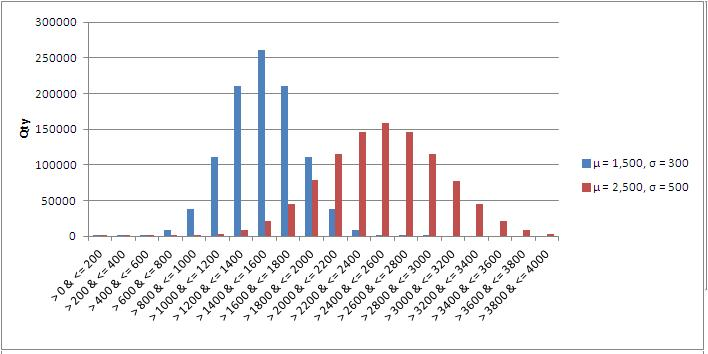# Monte Carlo Simulation

[ezcol_1half]

This Addin for Microsoft Excel aids in the performance of Monte Carlo analysis.

This Addin utilizes the Mersenne Twister algorithm to generate pseudo random numbers. The benefit to this is that the period is much longer than other methods. The native Excel VBA function, RND(), has a period of 16,777,216 numbers. After 16,777,216 pseudo random numbers are generated, the identical series of numbers repeats. The Mersenne Twister method has a much longer period of 2^(19937 − 1).

Functions are available to the Excel user to provide a random values whose distribution follows statistical functions. By specifying the parameters of the distribution the functions return random values whose frequency of occurrence follow the specified distribution. These functions are available to your VBA macros as well.

To demonstrate the capabilities, 1,000,000 random values were generated that follow a normal distribution with varying parameters. One set of parameters had a mean of 1,500 and a standard deviation of 300 and the other set had a mean of 2,500 and a standard deviation of 500. The results have been binned in the figure in increments of 200. The occurrences of randomly generated values follow the normal distribution.

The same process was followed for the Triangular, Beta-PERT, LogNormal, Weibull, Uniform, and Exponential distribution and the resulting graphics are provided.

### Product Benefits:

• Random number generator (Mersenne Twister) has much longer period and finer granularity over MS Office RAND() function.
• Functions are directly available to your workbook and custom VBA macros.
• Functions are compiled for speed, while late bound for maximum compatibility with MS Office products.

### Product Includes:

• Continuous Distributions:
• Exponential
• LogNormal
• Normal
• Beta
• Pert
• Triangle
• Uniform
• Weibull
• Distribution Specific Functions:
• PDF
• CDF
• Random Value

[/ezcol_1half]

[ezcol_1half_end]

## Normal Distribution## Triangular Distribution## Weibull Distribution## Uniform Distribution## Exponential Distribution## Beta-PERT Distribution